﻿ MMSE Partially Connected Hybrid Beam Forming in MIMO-OFDM Systems

# Journal of Telecommunications System & ManagementOpen Access

• Short Communication
• J Telecommun Syst Manage 2019, Vol 8(2): 2

# MMSE Partially Connected Hybrid Beam Forming in MIMO-OFDM Systems

Lenin SB1*, Tamilarasan N2 and Malarkkan S3
1Electronics and Communication, Pondicherry University, Muthialpet, Pondicherry, India
2Department of Electronics and Communication, Sri Indu College of Engineering and Technology, Telangana, India
3Mankula Vinayagar Institute of Technology, Pondicherry, India
*Corresponding Author: Lenin SB, Electronics and Communication, Sri Manakula Vinayagar Engineering College, Muthialpet, Pondicherry, India, Tel: +919488394885, Email: [email protected]

Received Date: May 30, 2019 / Accepted Date: Jun 10, 2019 / Published Date: Jun 17, 2019

### Abstract

Traditionally, Multi Input Multi Output-Orthogonal Frequency Division Multiplexing (MIMO-OFDM) technique is utilized to increase the diversity gain and to suppress the Inter Carrier Interference (ICI) to some extent. The problem of the existing system is to solve the sum rate maximization due to non-convexity. This paper aims to minimize the computational cost at the transceiver side and improve the spectral efficiency. This paper presents a minimum mean square error (MMSE) approach for partially connected Hybrid beam forming (HBF) weighted sum rate maximization.
Since the feedback of the BF system is more complicated, one-bit training is used to minimize the complications rather than a large bit training method. The paper gives more attention on BF technique for an IEEE802.11ad standard to transmit and receive the data. The proposed method focuses on the reduction of the complexity level, increases the spectral and power efficiency with an optimal number of radio frequency (RF) links. The MMSE estimation validates the results by comparing with various methods such as analog BF and fully digital in terms of loss calculation, sum
rate and normalized MSE. The analysis results are showed that MMSE attains optimal spectral and power efficiency.

Keywords: Energy efficiency; PC-HBF; ICI; MIMO-OFDM; MMSE

#### Introduction

In recent days, advanced wireless technologies have become important part in people’s lives because it makes the life very simple and more convenient. Presently, the number of users is significantly increased and it makes it difficult for limited bandwidth and need of high-speed communications in terms of Gbps. The advancements in technical development brought a challenge to wireless communications as the number of network devices is increased exponential. Although different approaches have been developed to achieve higher data rate, the availability of low bandwidth in current scenario restricts the channel capacity.

Due to the adverse channel features, beam forming (BF) techniques have been introduced in the MIMO systems for directing the beam with high array gain. With the help of smaller wavelength with higher frequencies, it is possible to carry more amounts of antennas in small areas and asymptotically attain the channel capability . The main aim of BF technique is to achieve the directionality of signal transmission (Direction of Arrival (DOA) and Angle of Arrival (AOA)) as well as power variations. It is utilized to minimize the interference as well as to increase diversity gain, antenna gain and array gain. An efficient beam selection method should provide high interference rejection. It is attained by the combination of antenna element in the phased array. The BF again can be classified into two ways: analog and digital. The analog BF is a simpler way, which receives the signal from various antenna arrays and every signal is co-phased as well as summed to maximize the gain. Here, the RF chain is restricted with a large number of antenna arrays are being used [2,3]. The limitations of analog BF are the difficult of controlling the signal amplitude and the low-resolution signal phase control . Next, the digital BF is not easier than analog technique, but, it sums all weights indicated by the complex weighing function and gives an output, using various radiating antennas which can simply decorrelates the signal and noise for users. It has some advantages like it can control multiple beams, amplitude as well as phase of the signals are easily controllable, and it supports for low rate sampling signals . It can provide a good solution but with the cost of high complexity.

Though two kinds of BF techniques offer various advantages, the package of many antennas comes with the sacrifice of energy utilization. It is impractical for implementing end to end RF chains for every antenna at a transmitter because of the limitations of energy and space. So, hybrid beam forming (HBF) techniques are introduced which make use of the benefits of analog BF and digital BF. Using HBF approaches, it is probable to get advantageous from the gain of a massive amount of antennas by the consumption of lower energy with less number of RF chains compared to antennas. In addition, HBF supports multi-stream and multiuser transmissions in easier manner. So, it is needed to increase the benefit of HBF with perfect channel estimation. Numerous methods have been presented on HBF integrating fully and partially connected structures based on heuristic ones to optimization algorithms . Partially connected HBF (PCHBF) model is a practical choice for a massive MIMO type of implementation as it needs only a limited number of lossy connections between RF chains and antenna elements . Two processing mechanisms are considered in the context of PCHBF namely full array and sub-array based ones. Channel state information (CSI) acquisition is one of the significant aspects of a (massive) MIMO system. Generally, a wireless channel has a nonzero coherence time in case of channel is considered as static. Some of the previous works on HBF with the consideration of perfect CSI is found in the literature [6-10]. In addition, the works [11,12] have considered both channel estimation and BF with hybrid architecture with no consideration of channel coherence time. The LMS algorithm is preferred  and is practically unsuitable for the wireless communication system. Power control or normalized LMS algorithm is the faster converging algorithm employed in mobile communication. The restricted capacity and the essential precision arithmetic in hardware have implementation problems and cause statistical instability in the normalized LMS algorithm. In multi-user massive MISO systems, lower complexity transmission methods, like zero forcing (ZF) is commonly used as it gives better results . Similarly, in a study , the linear minimum mean square error (LMMSE) estimator is used as the optimal linear estimator in the MSE sense. Regardless of the precise channel estimator, the LMMSE requires high computation cost, and it needs the prior knowledge of the channel covariance matrix and that of the noise level, which limits its implementation.

This paper mainly focused on the intention to minimize the complexity level of the model, increase the spectral and power efficiency with an optimal number of RF links. We present a minimum mean squared error (MMSE) based hybrid analog-digital channel estimator by considering the channel covariance matrix for perfect and imperfect path. It is presented for partially connected HBF to maximize the weighted sum rate. We utilize a one-bit feedback to estimate the sparse channel. So, the feedback overhead is reduced at the transmitter side and can easily estimate the channel conditions. Then, the MMSE estimation is validated by comparing its results with various methods such as analog BF and fully digital interms of loss calculation, sum rate and normalized MSE. The experimental analysis showed that MMSE attains optimal spectral and power efficiency. It also offers various benefits like reduced feedback overhead, low computation cost and power utilization.

The upcoming portion of the paper is arranged as follows: System model is explained in Section 2. The with partially connected Hybrid Beamforming-MMSE (PCHBF-MMSE) is presented in Section 3 and the results are validated in Section 4. Finally, the paper is concluded in below Section 5.

#### System Model

This section deals with Multi user-MIMO (MU-MIMO) system with PCHBF architecture with channel estimation technique shown in Figure 1. The architecture consists of BS with N antenna elements for serving multiple users equipped with 2or 4 antennas. Here, the CSI is present at the BS, which employs a hybrid model with NA RF chains. The PCHBF structure of the BS has the capability to control the amplitude as well as phase in the analog part. The antenna array of the BS is partitioned to N1 subarrays, with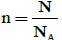antenna elements. The number of RF chains is assumed to be same as the number of users, i.e. NA=K (Figure 2) describes a typical MU-MIMO system, the transmitted signal is received by an antenna array each described by the AoD delay and AoA. Unlike the fast fading, the angles and delay are almost stationary over a considerable number of frames. The receiver estimates the channel and determines whether it is flat fading or frequency fading. Based on the analysis of mmwave, the channel has typically one to three clusters with random power allocation and random geographic area . The channel fading index (CI=0) for flat fading and CI=1 for frequency selective fading) is fed back to the transmitter. Sparsity structure and compressed sensing technique has been utilized to reduce the overhead of training data to estimate the channel . Instead of transmitting large amount of data as a feedback, the received symbol is quantized into one-bit value and then it sent back as feedback to the transmitter. Since the feedback of the BF system is more complicated, one-bit training is used to minimize the complications rather than a large bit training method. This scheme has more advantageous like it reduces the feedback overhead and it correctly estimates the AoA signal direction.

The antenna element of the serving station is divided into N1subarrays, with each antenna element n=N/NA and the RF chains a NA=K. The HBF structure is functioned with partially connected BF, where NRF is the number of RF chains, and a low-dimension digital beam former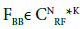For full array-based processing, where all data streams are conveyed to every RF chain, the digital precoder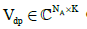can be defined as: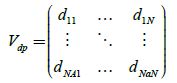(1)

For subarray based processing, every data stream is transmitted to only one RF chain, so that the digital precoder can be written as a diagonal matrix as Vdp=diag (daa,dbb,dcc,..,dkk) Next, the analog precode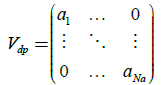(2)

Where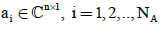is the analog precoder of the ith subarray.

Given Sk an Wk, for all k, let us first consider the fully digital BF matrix ν[νsw],νs=[ν1,s1,…,νk,wk] and νw=[ν1,w1,….νk,wk] both are the sub-matrices corresponding to strong and weak users, and νk,i is the beamformer for k user. Then, the received signal at user k can be indicated as: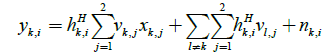(3)

The output of the hybrid beam former of the kth user is given as: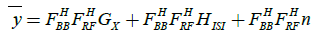(4)

Where FBB denotes digital beam signal, and FRF denotes analog beam signal, G=[g1,g2,…gk] represents the data-channel with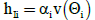corresponding to the link between the kth user and BS.

It is assumed that the incident interference contains different dominant rays, each one represents to an AoA. Then, the interference channel can be written aswhere scalar αi accounts for the path loss of hIi, and N×1 vector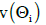is the phase response for the linear receiver array in the eqn. (5).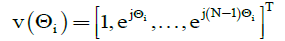(5)

The user uses the multi-path model for capturing the low rank and spatial correlation features of an mm Wave channel. The communication links between the users and the BS are exposed to interference arriving from M dominant AoA.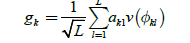(6)

Where L is the random number of independent and identically distributed (i.i.d.) routes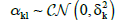indicates the i.i.d path gain respective to the user index k and path index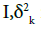is the kth channel’s average attenuation.

#### Channel Estimation for PCHBF-MMSE

Given Sk and Wk, for all k, let us first consider the fully digital BF ν[νS, νW],VS=[V1,S2,…,Vk,Sk and VW=[V1,w2,..,Vk,wk] are the sub matrices corresponding to strong and weak users, and νk,i is the beamformer for k user. Then, the received signal at user , indicates in the equation: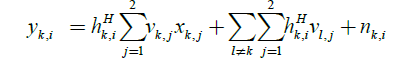(7)

The inter-pair interference at user (k,i) is given as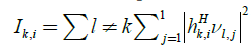and the attainable rates of user (k,sk) and (k,wk) are represented as: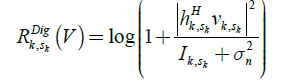(8)

And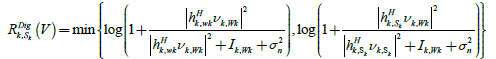(9)

The weighted sum rate maximization can be formulated as: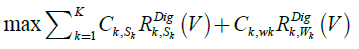(10)

Subject to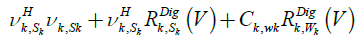(11)

For establishing the relationship between MMSE and SINR, let us first define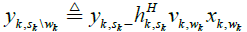as the efficient received signal at Sk after cancellation of the signal intended for Wk, and assume the estimate: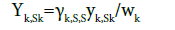(12)

Where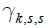is selected for the minimization of MSE.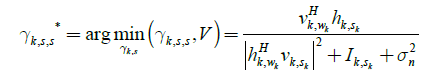(13)

and the resulting MSE is: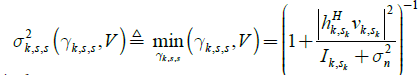(14)

And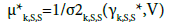(15)

The MSE of the estimate on xk,wk at the strong and weak users using observations are yk,Sk and yk,wk respectively. Therefore, the achievable rate of user k,Wk can be expressed and the optimal value for μk,s,w and μk,w,w are given by: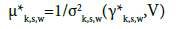(16)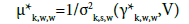(17)

The optimal γk,s,w and γk,w,w are given by: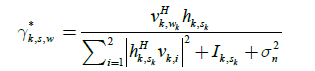(18)

And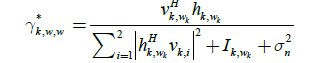(19)

We have validated the analytical expressions by performing numerical and extensive Monte Carlo simulations. The eigen values of the covariance output signal with the covariance of the error signal is describe the information in the system. The achievable rate of user k can be expressed as: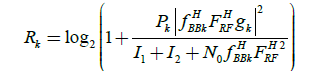(20)

Rk is gives the maximum data rate of the receiver. The achievable maximum sum-rate of the multi user system is attained by: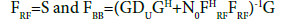(21)

where G denotes the effective channel BF.

Performance evaluation

Parameter settings: To validate the performance analysis of the PCHBF-MMSE channel estimation methods, a comparison is made with analog BF and fully digital BF. A set of parameters used for simulation are provided in Table 1.

Parameter Value
Nt, no. of antennas arrays 64
Number of RF chains (L) 3
The number of clusters (K) 3
The number of channel paths (M) 4
Distance 100, 500
Estimation MMSE

Table 1: Parameter setup.

#### Results

This section shows the comparative analysis of analog BF (ABF), fully digital (FD), and PCHBF-MMSE with 4 and 8 number of RF links. The metrics used to analyze the results are MIMO-OFDM with different subcarriers, sum rate, average sum rate and loss calculation. In this section, we provide numerical simulations to demonstrate the effectiveness of the proposed MMSE scheme. Figure 3 shows the comparative results of varying number of sub carriers with varying number of antennas in PCHBF. From the figure, it is clearly shown that the poor performance is attained for lower number of SC with less number of antennas. Similarly, the performance is significantly improved for higher number of SC with more number of antennas.

It can be seen from the figure that poor performance is obtained with 64SC with 32*2 antennas and the performance is slightly increased when the 64SC with 32*2 antennas is changed to 128SC with 64*2 antennas. At the same time, it is observed that the performance is found to be even better for 64 SC with 32*4 antennas compared to 64SC with 32*2 and 128SC with 32*2. Likewise, the maximum performance is achieved for the increased number of 128 SC with 64*4 antennas. These values imply that better performance is achieved for increased number of sub carriers with more number of antennas.

The performance analysis is done based on antennas used in the base station and the results compared between fully digital and PCHBF depends on number of antennas used in the system. The sum rate performance is analyzed for N number of antennas and is showed in Figure 5 and the results are compared for different methods in terms of SNR. Several observations are made and it is found that the PCHBF - MMSE gives better solution which exhibits a linear increasing in user SNR signal, here sum rate is increased and interference is reduced simultaneously. From this figure, it is clear that the fully digital achieves better performance whereas the analog BF with 4 RF links. In addition, it is noted that the fully digital with 4 RF links performance seems better than analog BF with 8 RF links and not greater than partially connected with 8 RF links. These values indicate that the sum rate can be increased with an increase number of antenna and RF links.

Next, an in depth analysis in terms of path loss calculation is shown in Figure 6. Here, a comparison is made between the PCHBF and fully digital with 4 and 8 RF links. From the figure, it is clear that the loss is only around 3dB for partially connected with 4 RF links. At the same time, it is noted that almost similar performance is attained by fully digital with 4 RF links. Similarly, for the 8 RF links, the signal loss is much lower than the analog BF by approximately 0.5 to 1dB. Interestingly, the fully digital BF with 8 RF links attains minimum loss but with the cost of high computation complexity and power consumption.

In Figure 7, a comparison is made between analog BF, partially connected MMSE and fully digital BF interms of a normalized MSE value. From this figure, it is clear that analog BF attained higher MSE compared proposed MMSE and fully digital. At the same time, it is noted that MMSE achieved lower MSE and found to be efficient compared to analog BF. But, the fully digital showed better results compared to MMSE. These results showed that the MMSE achieves a better tradeoff between computational complexity and efficiency (Figure 8).

#### Conclusion

To mitigate the interferences in MIMO-OFDM systems, PCHBF gives a significantly improved performance than analog BF. An extensive set of experimental analysis is carried out to analyze the efficiency of the PCHBF-MMSE for MIMO-OFDM systems. It is investigated with varying number of antennas, sub carriers and RF links. The results indicated that the efficiency of the applied model is increased with an increased number of subcarriers, antennas as well as RF links. It is also noted that PCHBF is found to be better than analog BF but not than fully digital BF. The experimental results showed that the MMSE achieves a better tradeoff between computational complexity and efficiency.

#### References

Citation: Lenin SB (2019) MMSE Partially Connected Hybrid Beam Forming in MIMO-OFDM Systems. J Telecommun Syst Manage 8: 181.

Copyright: © 2019 Lenin SB. This is an open-access article distributed under the terms of the Creative Commons Attribution License, which permits unrestricted use, distribution, and reproduction in any medium, provided the original author and source are credited.

Select your language of interest to view the total content in your interested language

##### Recommended Journals
Viewmore
###### Article Usage
• Total views: 404
• [From(publication date): 0-0 - Dec 15, 2019]
• Breakdown by view type
• HTML page views: 366Can't read the image? click here to refresh